Search results

# Pointers in ASP.NET Core Linear Gauge control

15 Oct 2021 / 5 minutes to read

Pointers are used to indicate values on an axis. The value of the pointer can be modified using the `Value` property in `e-lineargauge-pointer`.

tagHelper
``````@using Syncfusion.EJ2.LinearGauge

<ejs-lineargauge id="linear">
<e-lineargauge-axes>
<e-lineargauge-axis>
<e-lineargauge-pointers>
<e-lineargauge-pointer Value="80"></e-lineargauge-pointer>
</e-lineargauge-pointers>
</e-lineargauge-axis>
</e-lineargauge-axes>
</ejs-lineargauge>``````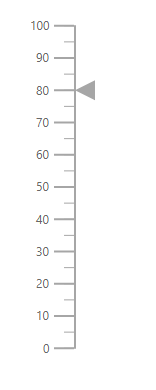## Types of pointer

The Linear Gauge supports the following types of pointers:

• Bar
• Marker

The type of pointer can be modified by using the `Type` property in `e-lineargauge-pointer`.

### Marker pointer

A marker pointer is a shape that can be used to mark the pointer value in the Linear Gauge.

Types of marker shapes

By default, the marker shape for the pointer is InvertedTriangle. To change the shape of the pointer, use the `MarkerType` property in `e-lineargauge-pointer`. The following marker types are available in Linear Gauge.

• Circle
• Rectangle
• Triangle
• InvertedTriangle
• Diamond
• Image

An image can be rendered instead of rendering a shape as a pointer. It can be achieved by setting the `MarkerType` property to Image and setting the source URL of image to `ImageUrl` property in `e-lineargauge-pointer`.

tagHelper
``````@using Syncfusion.EJ2.LinearGauge

<ejs-lineargauge id="linear">
<e-lineargauge-axes>
<e-lineargauge-axis>
<e-lineargauge-pointers>
<e-lineargauge-pointer Type="Marker" MarkerType="Circle" Value="60"></e-lineargauge-pointer>
</e-lineargauge-pointers>
</e-lineargauge-axis>
</e-lineargauge-axes>
</ejs-lineargauge>``````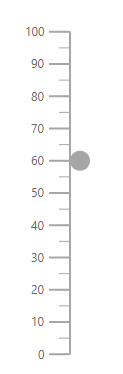Marker pointer customization

The marker pointer can be customized using the following properties and class.

tagHelper
``````@using Syncfusion.EJ2;
<script>
var axis = args.gauge.axes;
axis.pointers = [{
value: 80,
markerType: 'Circle',
height: 15,
width: 15,
color: '#cd41f4'
}]
}
</script>
=======
@using Syncfusion.EJ2.LinearGauge

<ejs-lineargauge id="linear">
<e-lineargauge-axes>
<e-lineargauge-axis>
<e-lineargauge-pointers>
<e-lineargauge-pointer Type="Marker" Height="15" Width="15" MarkerType="Circle" Value="60" Position="Outside" Color="#cd41f4"></e-lineargauge-pointer>
</e-lineargauge-pointers>
</e-lineargauge-axis>
</e-lineargauge-axes>
</ejs-lineargauge>``````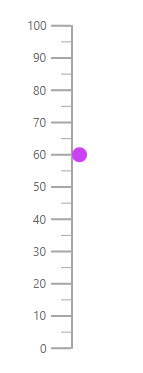### Bar pointer

The bar pointer is used to track the axis value. The bar pointer starts from the beginning of the gauge and ends at the pointer value. To enable bar pointer set the `Type` property in `e-lineargauge-pointer` as Bar.

tagHelper
``````@using Syncfusion.EJ2.LinearGauge

<ejs-lineargauge id="linear">
<e-lineargauge-axes>
<e-lineargauge-axis>
<e-lineargauge-pointers>
<e-lineargauge-pointer Type="Bar" Value="60"></e-lineargauge-pointer>
</e-lineargauge-pointers>
</e-lineargauge-axis>
</e-lineargauge-axes>
</ejs-lineargauge>``````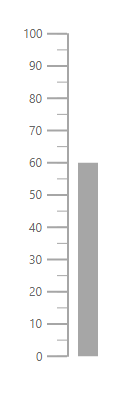Bar pointer customization

The bar pointer can be customized using following properties and class.

The Placement property is not applicable for the bar pointer.

tagHelper
``````@using Syncfusion.EJ2.LinearGauge

<ejs-lineargauge id="linear">
<e-lineargauge-axes>
<e-lineargauge-axis>
<e-lineargauge-pointers>
<e-lineargauge-pointer Type="Bar" Width="20" Value="60" Color="#f44141"></e-lineargauge-pointer>
</e-lineargauge-pointers>
</e-lineargauge-axis>
</e-lineargauge-axes>
</ejs-lineargauge>``````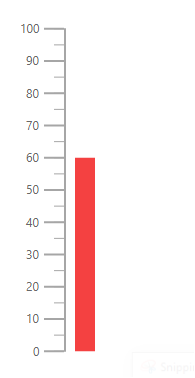## Multiple pointers

Multiple pointers can be added to the Linear Gauge by adding multiple `e-lineargauge-pointer` in the `e-lineargauge-pointer` and customization for the pointers can be done with `e-lineargauge-pointer`.

tagHelper
``````@using Syncfusion.EJ2.LinearGauge

<ejs-lineargauge id="linear">
<e-lineargauge-axes>
<e-lineargauge-axis>
<e-lineargauge-pointers>
<e-lineargauge-pointer Value="80"></e-lineargauge-pointer>
<e-lineargauge-pointer Value="30" MarkerType="Circle"></e-lineargauge-pointer>
<e-lineargauge-pointer Value="60" MarkerType="Diamond"></e-lineargauge-pointer>
</e-lineargauge-pointers>
</e-lineargauge-axis>
</e-lineargauge-axes>
</ejs-lineargauge>``````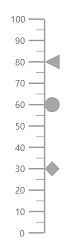## Pointer animation

Pointer is animated on loading the gauge. This can be handled using the `AnimationDuration` property. The duration of the animation can be specified in milliseconds.

tagHelper
``````@using Syncfusion.EJ2.LinearGauge

<ejs-lineargauge id="linear">
<e-lineargauge-axes>
<e-lineargauge-axis>
<e-lineargauge-pointers>
<e-lineargauge-pointer Value="60" AnimationDuration="1000"></e-lineargauge-pointer>
</e-lineargauge-pointers>
</e-lineargauge-axis>
</e-lineargauge-axes>
</ejs-lineargauge>``````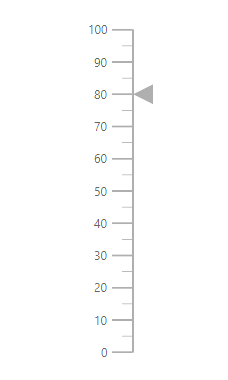Gradient support allows the addition of multiple colors in the pointers of the Linear Gauge. The following gradient types are supported in the Linear Gauge.

Using linear gradient, colors will be applied in a linear progression. The start value of the linear gradient can be set using the `StartValue` property. The end value of the linear gradient will be set using the `EndValue` property. The color stop values such as `Color`, `Opacity`, and `Offset` are set using `ColorStop` property.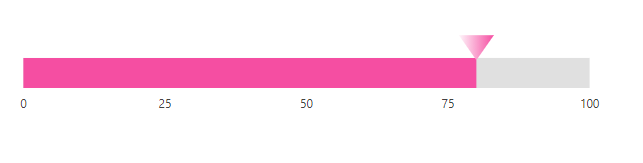Using radial gradient, colors will be applied in circular progression. The inner circle position of the radial gradient will be set using the `InnerPosition` property. The outer circle position of the radial gradient can be set using the `OuterPosition` property. The color stop values such as `Color`, `Opacity`, and `Offset` are set using `ColorStop` property.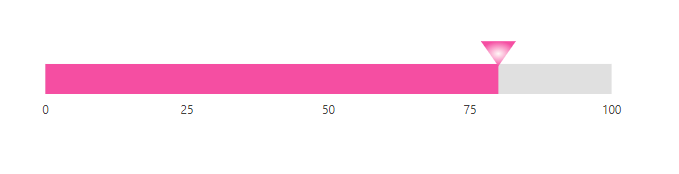If we set both gradients, only the linear gradient gets rendered. If we set the `StartValue` and `EndValue` of the `LinearGradient` as empty strings, then the radial gradient gets rendered in the pointer of the Linear Gauge.